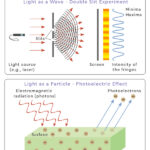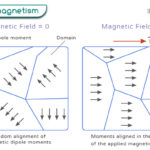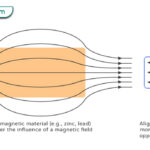Home / Physics / Convection

# Convection

Convection is the heat transfer process from one region to another due to the movement of fluids like air or water. The initial heat transfer occurs through conduction, but the primary transfer occurs through convection. It is the dominant form of heat transfer in liquids and gases.

## Types of Convection

Convection is of two types – natural and forced.

Natural convection is due to buoyancy and the buoyant force acting on the fluid. For natural convection to occur, there has to be a temperature difference across the fluid. Examples of natural convection include sea breeze and land breeze.

Forced convection occurs when an external agent, like a fan or pump, transports the fluid. The effect is similar to natural convection. Examples of forced convection include a convection oven, air conditioner, car radiator, and water heater.

## How Does Heat Transfer by Convection Occur

A space heater is an excellent example of understanding natural convection. It heats the air at the bottom of a room. As a result, the kinetic energy of the particles increases, and the air expands. The air becomes less dense and rises to the top. Then, it cools down, reduces its volume, and becomes denser. The cold air sinks and reaches the bottom, where it heats up. This process repeats itself and forms a cycle known as convection currents.

## Equation

Heat transfer in convection typically occurs between a surface and a fluid, where the fluid carries the heat generated by the surface. The equation for heat flow is $$Q = h_C A (T_S – T_f)$$

Where

Q: Heat transferred per unit time

hc: Convective heat transfer coefficient

TS: Temperature of the surface

Tf: Temperature of the fluid

The unit for the convective heat transfer coefficient is Wm2K1 It depends on the physical properties of the surface and the fluid, like surface geometry, bulk fluid velocity, and the nature of fluid motion.

## FAQs

Q.1. How do convection currents cause mountains and earthquakes?

Ans. Convection currents in the Earth’s mantle cause the movement of tectonic plates in the crust, resulting in mountains and earthquakes.

Article was last reviewed on Monday, January 2, 2023

### Related articlesWave-Particle DualityPhotoelectric EffectFerromagnetismDiamagnetism